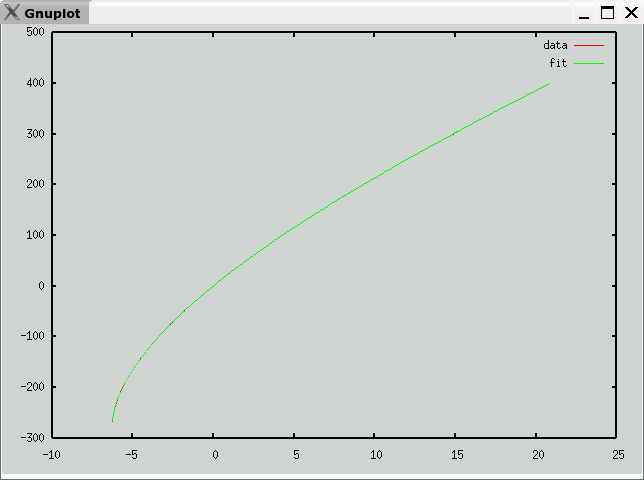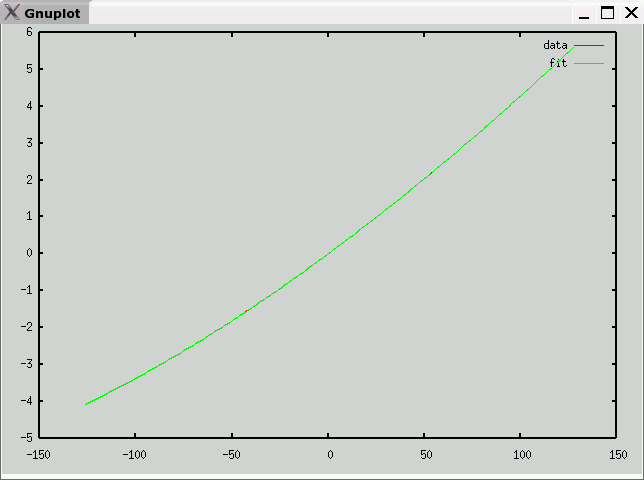# Type T Thermocouple Data and Polynomial

• Description
Cu / Cu-Ni
• Data source
National Institute of Science and Technology (NIST)
• Raw Data
• Octave format
• Curve fit
• mV -> temperature (C)
e is maximum error (in degrees C)
v1 andf v2 partition the domain into three segments, each with a polynomial fit
c is vector of coefficients: a10, a9, ... a0
Note: Each column of c corresponds to one range (<v1,v1 to v2, >v2)

`e = 0.26247v1 = -1.7850v2 = 2.5560c =   -8.5687e-02    1.5143e-04  -5.4487e-11   -3.3559e+00    3.3541e-05   6.4923e-09   -5.8195e+01   -3.7206e-03   -3.3847e-07   -5.8795e+02    3.2654e-03   1.0239e-05   -3.8295e+03    2.2672e-02  -2.0117e-04   -1.6790e+04   -2.2290e-02   2.7236e-03   -5.0151e+04   -6.9454e-02   -2.6371e-02   -1.0070e+05    1.1688e-01   1.9264e-01   -1.3004e+05   -6.7161e-01   -1.3081e+00   -9.7452e+04    2.5826e+01   2.7040e+01   -3.2203e+04   -2.4547e-03   -9.0066e-01 `• temperature -> mV (range -127C-127C)
e is maximum error (in mV)
c is vector of coefficients: a10, a9, ... a0

`e = 0.00088971c =   -1.1498e-22    7.1847e-21    5.3169e-18   -2.8841e-16   -9.5145e-14    4.0271e-12    8.6066e-10   -5.7469e-08    4.1754e-05    3.8675e-02   -2.5313e-04 `Previous page: Type S